Courses

# Discrete Simulation Models - 3 Civil Engineering (CE) Notes | EduRev

## Civil Engineering (CE) : Discrete Simulation Models - 3 Civil Engineering (CE) Notes | EduRev

The document Discrete Simulation Models - 3 Civil Engineering (CE) Notes | EduRev is a part of Civil Engineering (CE) category.
All you need of Civil Engineering (CE) at this link: Civil Engineering (CE)

Numerical Example

Assume a two-lane road divided into nine cells in each of its lane. In first lane vehicles are present in first (1), third (1), fourth (2), eight (1) cells and in second lane vehicles are present in fifth (1) and sixth (1) cells. The numbers in the brackets indicate the present velocities of the respective velocities. Apply the lane changing rules and determine which vehicles fulfilled the lane changing requirements.

Solution

Initially the solution starts with the acceleration stage of the vehicles, where the vehicles are applied with the acceleration rule (equation. 20.5). In the acceleration stage, a single unit increase in every vehicle, which possesses a velocity less than the maximum velocity. So stage of the vehicles after the acceleration are shown in the Fig. 20:5. Lane changing is required for L1(1), L1(2), L2(1) where “L1(1)” indicates number in the subscript as its lane number and the superscript as it vehicle number in respective lanes.

Rule 1: ∆Xp p (n) < V (n) + 1 The first vehicle has a velocity two and the gap ahead of it in its current lane is 2 so according to the rule (velocity +1 > gap). Therefore, the vehicle satisfied the rule so that it can change the lane. L1(1) = (2 < 2 + 1) . . . satisfied. Similarly checking for all the other vehicles. L1(2) = (1 < 2 + 1) . . . satisfied, L2(1) = (1 < 2 + 1) . . . satisfied. The term gap is generally referred in two different ways, where it is explained as the distance between bumper to bumper of the vehicles. The other way to state, the term gap is the number of empty cells in front of a vehicle”. Here in the present discussion it is taken as the earlier one but anyways it depends on the reader of choosing it where a slight modification (i.e., addition of ± 1on the other side of the equations).

Rule 2 : (∆Xf o (n) > V (n) + 1 ) (as per the rule 10) The velocity of the first vehicle is two and the gap in the target lane ahead of it is four so the rule ( gap ( target lane ) > velocity + one ) is satisfied for the first vehicle. L1(1) = (4 > 2 + 1) . . . satisfied. Similarly checking for other vehicles also will obtain the following results. L1(2) = (2 6> 2 + 1) . . . rejected, and L2(1) = (34 6> 2 + 1) . . . rejected.

Rule 3: No collision of vehicles is observed as per the pattern given.

Rule 4: (∆Xb o (n) > Vmax ) (as per the rule 11) The maximum velocity of any vehicle is given as four and the gap behind the first vehicle in the target lane is five which is greater than the maximum velocity so the vehicle satisfied the rule and subjected to lane change. L1(1) = (5 > 4). Therefore the first vehicle in the first lane satisfied all the four rules.

Limitations

In real world traffic the vehicles dont have unique velocities but it was an assumption in the model. So the vehicles are further divided into two types of different Vmax, namely Vfmax, Vsmax, corresponding to fast vehicles and a slow vehicles. Introduction of “symmetric two lane model” for inhomogeneous traffic.

Extensions

There are two types

Sequential update : This updating procedure considers each cell in the lattice one at a time. If all cells are considered consecutively, two updating directions are possible, left-to-right and right-to-left. There is also a third possibility, called random sequential update. Under this scheme and with N particles in the lattice, each time step is divided in N smaller sub steps.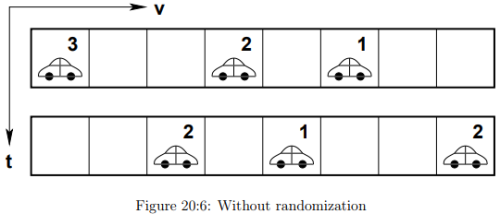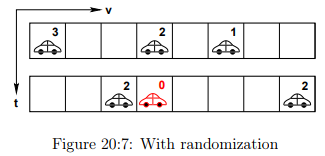At each of these sub steps, a random cell (or vehicle) is chosen and the CA rules are applied to it.

Parallel update: This type of update is the classic update procedure generally used in all the models. For a parallel update, all cells in the system are updated in one and the same time step. Compared to a sequential updating procedure, this one is computationally more efficient (note that it is equivalent to a left-to-right sequential update).

Effect of Randomization

The Fig. 20:6 shows the updation without randomization and the Fig. 20:7 is with randomization where an extra deceleration is observed in second vehicle and then updation.

Totally asymmetric simple exclusion principle

The simple exclusion process is a simplified well-known particle transport model from nonequilibrium statistical mechanics, defined on a one-dimensional lattice. In the case of open boundary conditions (i.e., the bottleneck scenario), particles enter the system from the left side at an entry rate α , move through the lattice, and leave it at an exit rate β. The term ‘simple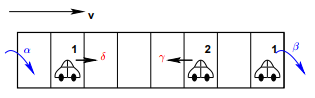Figure 20:8: Total asymmetric exclusion process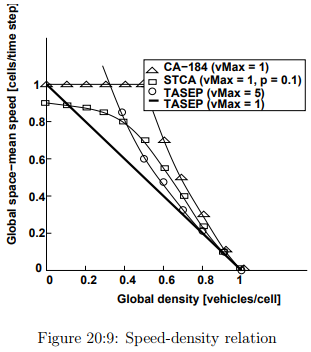exclusion’ refers to the fact that a cell in the lattice can only be empty, or occupied by one particle. When moving through the lattice, particles move one cell to the left with probability, and one cell to the right with probability γ. When γ = δ, the process is called the symmetric simple exclusion process (SSEP); if γ = δ then it is called the asymmetric simple exclusion process (ASEP). Finally, if we set γ = 0 and δ = 1, the system is called the totally asymmetric simple exclusion process (TASEP). If we consider the TASEP as a TCA model, then all vehicles move with Vmax = 1 cell/time step to their direct right-neighbouring cell, on the condition that this cell is empty. The process is shown in the below Fig. 20:8.

Comparisons

The above Fig. 20:9 gives a differentiation between four types of models and interestingly it is observed that the TASEP with Vmax = 1 has a trend of the Greenshield model and following a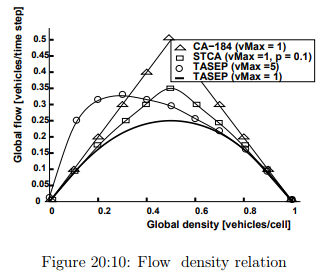linearity in the speed-density relation. The same trend is also observed in the below Fig. 20:10 flow density curve.

Offer running on EduRev: Apply code STAYHOME200 to get INR 200 off on our premium plan EduRev Infinity!

,

,

,

,

,

,

,

,

,

,

,

,

,

,

,

,

,

,

,

,

,

;